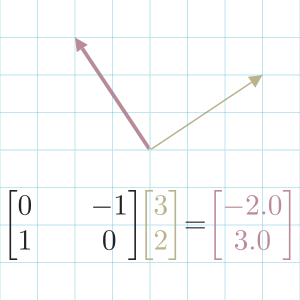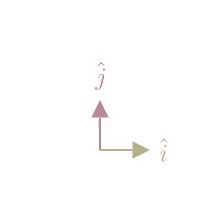## Matrix TransformationWe can think of a matrix as a transformation of a Vector or all vectors in space.

When we take the product of a matrix and a vector, we are transforming the vector.

A transformation is another word for a function: it takes in some inputs (a vector) and returns some output (a transformed vector).

For example, we can rotate a vector $\begin{bmatrix}x \\ y\end{bmatrix}$ some angle $\theta$ about the origin using a Rotational Matrix.

$\begin{bmatrix}\text{x*} \\ \text{y*} \end{bmatrix} = \begin{bmatrix}\cos\theta && \sin\theta \\ -\sin\theta && \cos\theta\end{bmatrix} \begin{bmatrix}x \\ y\end{bmatrix}$

In this example, we perform a 90° rotation of vector $\begin{bmatrix}2 \\ 2\end{bmatrix}$.To describe a transformation as a matrix, we only need to record where the Basis Vectors land as columns of a new matrix: $\begin{bmatrix}\color{red}{a} && \color{green}{b} \\ \color{red}{c} && \color{green}{d}\end{bmatrix}$

For example, a Shear Transformation keeps the $\hat{i}$ basis vector fixed, and slants the $\hat{j}$ basis vector. We can record that as: $\begin{bmatrix}\color{red}{1} && \color{green}{1} \\ \color{red}{0} && \color{green}{2}\end{bmatrix}$A matrix transformation is always linear in that it keeps all gridlines in space are parallel and evenly spaced.

3Blue1Brown (2016)

Dye et al. (2018)

Image processing is a use case for matrix transformations.

Since we represent an image as a $m \ x \ n$ grid of pixels, we can treat the position of each pixel as a vector, then perform a transform of each position vector to transform the entire image.

In this example, I rotate an image using the rotational matrix above.

• Create a new matrix that's the maximum possible width and height.
• Convert each position into a vector.
• Convert each position vector, so it's a distance from the center, not top-left.
• Rotate each position.
• Revert convert using a new image size.

Note that we end up with some empty pixels in a 45° rotation. These occur because some of the transformed coordinates are floating-point numbers. When they get rounded into integer positions, some of the pixels get excluded. There are many strategies to deal with this, but that's for another article.

Agrawal (202)

#### References

Gautam Agrawal. Rotating Image By Any Angle Using Only NumPy. September 202. URL: https://gautamnagrawal.medium.com/rotating-image-by-any-angle-shear-transformation-using-only-numpy-d28d16eb5076.

David Dye, Sam Cooper, and Freddie Page. Mathematics for Machine Learning: Linear Algebra - Home. 2018. URL: https://www.coursera.org/learn/linear-algebra-machine-learning/home/welcome.

3Blue1Brown. Linear transformations and matrices. August 2016. URL: https://www.youtube.com/watch?v=k7RM-ot2NWY.